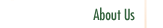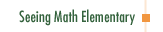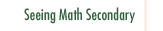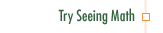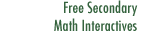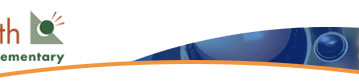Pre-Algebra: Patterns and Functions

In Pre-Algebra: Patterns and Functions, a fourth grade class explores the concept of function by analyzing a sequence of shapes. Using tiles, pictures, T-charts, and linear graphs students try to figure out a growth rule, or function, that allows them to make the next member of the sequence and predict how many tiles it has.

Participants in this course:

• Examine the development of student algebraic thought, centering on the concept of functions.
• Use and informally define the following terms (as applied to the study of functions): function, unknown, variable, equation, formula, algebraic expression, iteration, local property, and global property.
• Explain how a function is different from its representation through a pattern, T-chart, graph, or expression in algebraic symbols.
• Examine ways to represent functions, including sequential patterns, T-charts, number recipes or expressions, coordinate graphs, and the use of symbols for variables. How do the different ways to represent functions help students make the conceptual leap from the particular to the general, moving from "add four each time" to "this function works for all the stages of this pattern" to, finally, "functions are mathematical objects that describe many different patterns and situations"?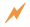Click to Get Seeing Math™ ElementaryCourse Listing Effective Questioning in the Mathematics Classroom Foundations of EffectiveMathematics Teaching Formative Assessment in the Mathematics Classroom Number & Operations: Division with Remainders Number & Operations: The Magnitude of Fractions Number & Operations: Broken Calculator Geometry: 2D and 3D Figures Geometry: Calculating Area of a Triangle Data Analysis and Probability: Using Data to Make Predictions Data Analysis and Probability: Measures of Center Pre-Algebra: Pan Balance Equations Pre-Algebra: Patterns and Functions